c# fir 滤波器_fir低通滤波c# - CSDN
• 最近需要用到Fir滤波器，在网上也看了不少资料，发现一个稍微能用的（https://blog.csdn.net/BIGFatming/article/details/92386914），主要代码也是直接copy的，但是在使用过程中发现，三角窗的实现好像不对，而且只...
• 最近需要用到Fir滤波器，在网上也看了不少资料，发现一个稍微能用的（https://blog.csdn.net/BIGFatming/article/details/92386914），主要代码也是直接copy的，但是在使用过程中发现，三角窗的实现好像不对，而且只...
最近需要用到Fir滤波器，在网上也看了不少资料，发现一个稍微能用的（https://blog.csdn.net/BIGFatming/article/details/92386914），主要代码也是直接copy的，但是在使用过程中发现，三角窗的实现好像不对，而且只实现了低通的，根据该文章内容，我自己重写了一个，包括三角窗函数的实现，添加了高通、带通、带阻的实现。环境Visual Studio2015，c#。

1、如下图（最下面的曲线），使用网友的三角函数实现不对；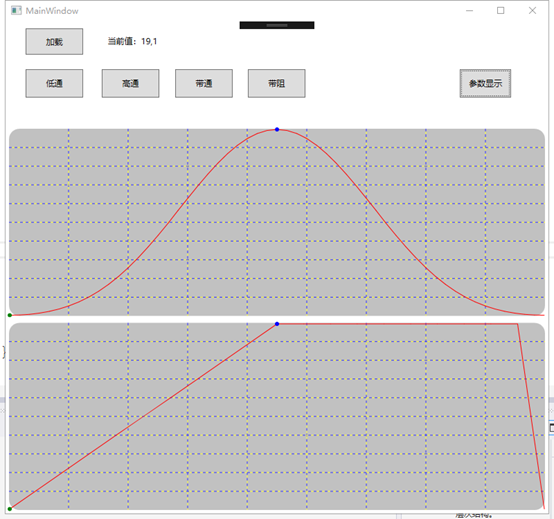2、据此更改了相关代码；

public class Bartlett
{
public int N = 0;
public Bartlett(double Wp, double Ws)
{
int i;
double n = (2.1 * 2 * Math.PI) / (Ws - Wp);
for (i = 0; i < n; i++) ;
N = i;
}

public double[] GetWin()
{
int n;
double[] wd = new double[N];
for (n = 0; n < N; n++)
{
if (n <= ((N - 1) / 2))
{
wd[n] = (double)(2 * (double)n / ((double)N - 1));
}
if (n > ((N - 1) / 2))
{
wd[n] = 2 * ((double)N - 1 - n) / ((double)N - 1);
}
}
return wd;
}
}


3、下图为Blackman和三角函数的h(n)；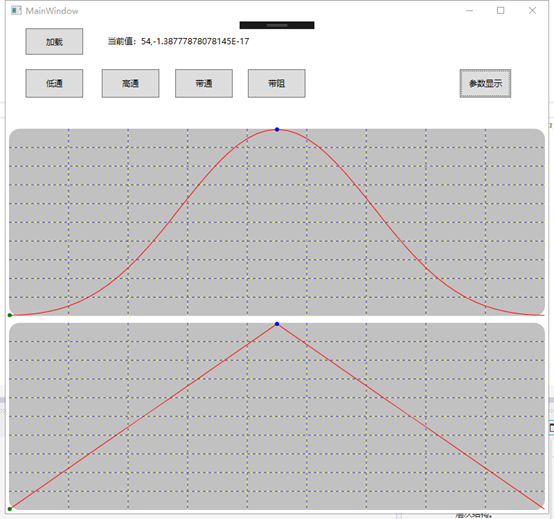4、下图为Hanning和Hamming窗的h(n)；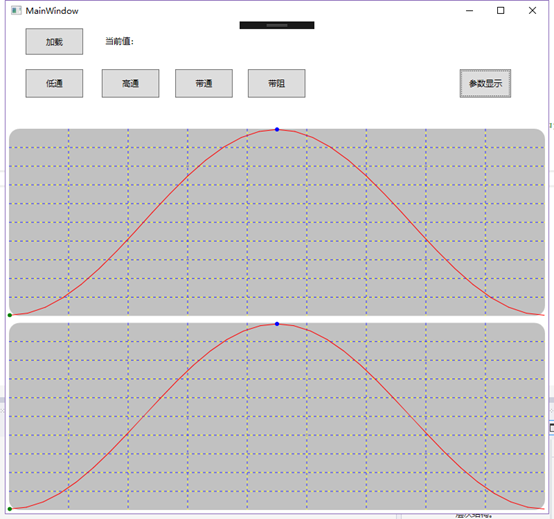5、由于看到的资料只提供了低通的实现方式，根据《数字信号处理理论、算法与实现（第三版）》实现了对应的高通、带通、带阻的实现；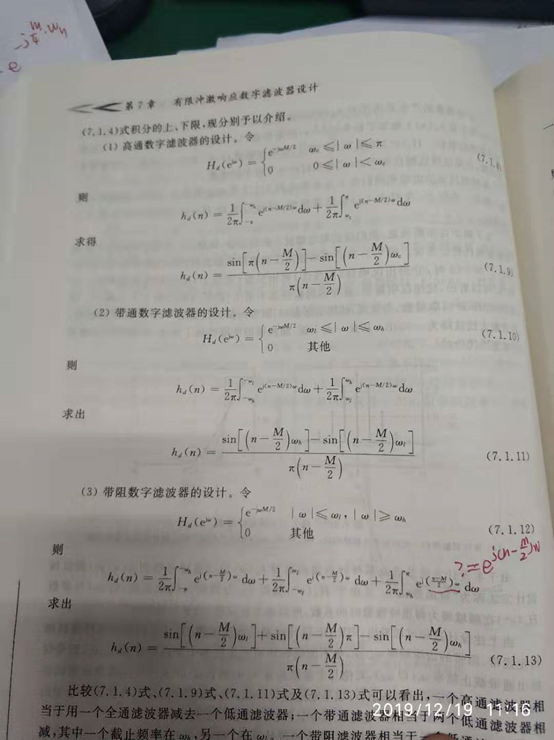6、高通

public double[] GetHigh()
{
double[] hd = new double[N];
for (int n = 0; n < N; n++)
{
double numerator = Math.Sin(Math.PI * (n - alpha)) - Math.Sin((n - alpha) * Wc);
double denominator = Math.PI * (n - alpha);
if (n == alpha)
{
hd[n] = (Math.PI - Wc) / Math.PI;
}
else
{
hd[n] = numerator / denominator;
}
}
return hd;
}


7、带通

public double[] GetDaiTong(double Wl, double Wh)
{
double[] hd = new double[N];
for (int n = 0; n < N; n++)
{
double numerator = Math.Sin((n - alpha) * Wh) - Math.Sin((n - alpha) * Wl);
double denominator = Math.PI * (n - alpha);
if (n == alpha)
{
hd[n] = (Wh - Wl) / Math.PI;
}
else
{
hd[n] = numerator / denominator;
}
}
return hd;
}


8、带阻，这里设计时，对于求n=alpha时的hd[n]值不是很确定，上图中用红笔注释的地方（为下面代码实现的内容）。

注：能力有限，在分析原始算法时，我是无法求解n=alpha的值或表达式（有人知道原始算法如何求解的话请留言，3Q），故带阻的实现可能在n=alpha点时是错的。【后期再进行研究，及时更新】。

public double[] GetDaiZu(double Wl, double Wh)
{
double[] hd = new double[N];
for (int n = 0; n < N; n++)
{
double numerator = Math.Sin((n - alpha) * Wl) + Math.Sin(Math.PI * (n - alpha)) - Math.Sin((n - alpha) * Wh);
double denominator = Math.PI * ((double)n - alpha);
if (n == alpha)
{
hd[n] = (Math.PI - Wh) / Math.PI + Wl / Math.PI;
}
else
{
hd[n] = numerator / denominator;
}
}
return hd;
}


9、测试：（测试代码均使用的hanning窗，测试低通、高通、带通与带阻功能）

9.1、原始信号参数为：Fs=1000，采样点数：2048，原始数据包含两个频率点数据5Hz和20Hz。Matlab代码：

f1=5;f2=20;f3=15;
t=0:0.001:2.049;
y=2*cos(2*pi*f1*t)+2*sin(2*pi*f2*t);
plot(t,y);
fid=fopen('test1.txt','wt');
fprintf(fid,'%d,',y);
fclose(fid);


9.2、原始信号及频谱，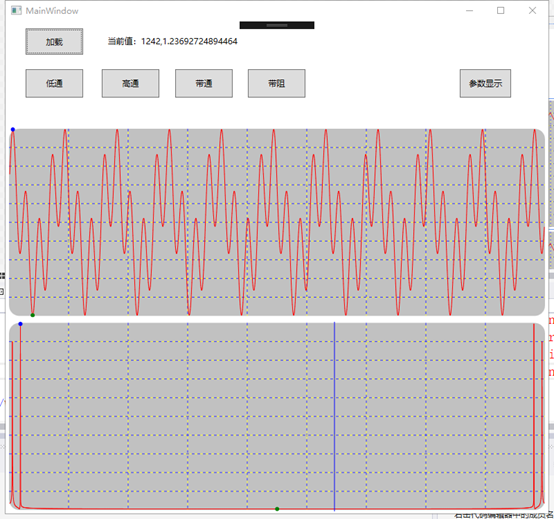9.3、低通滤波，Wp=0.02 * Math.PI，Ws=0.03 * Math.PI（分别对应Wp=10Hz，Ws=15Hz）

注：由于给入的频率为归一化后的，需要计算（(Fs/2)*Wp=fp，截止频率为fc【Wc为归一化频率】）；相位延迟：(1/Fs)*(N-1)/2；滤波器阶数：N-1(N为窗长度)【这里不确定，网上查到有这个说法，有大神知道的话可以探讨一下】。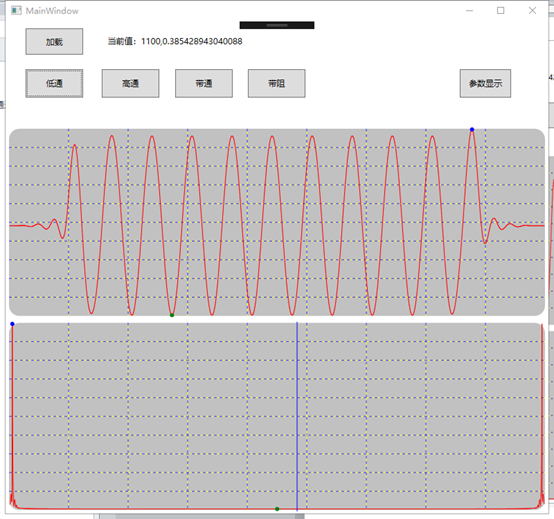9.4、高通滤波，参数与低通一致，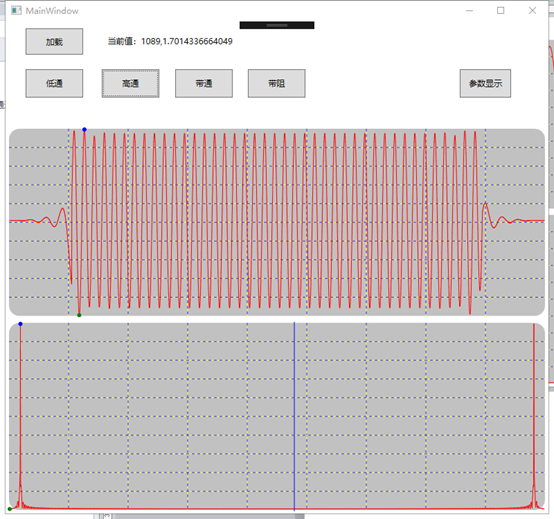9.5、带通滤波，带通频率设置为【0.03 * Math.PI, 0.05 * Math.PI】（即为15Hz~25Hz，通带）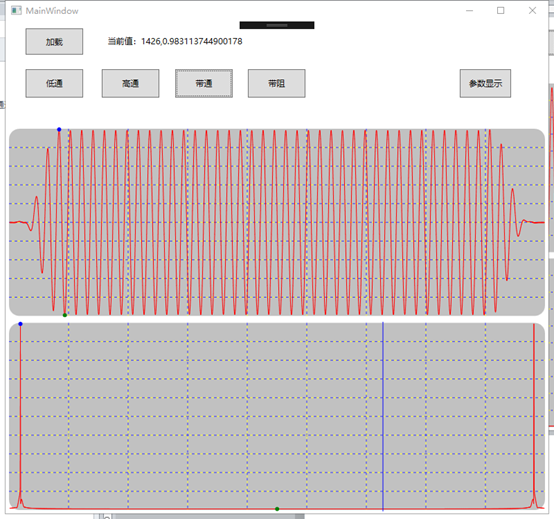9.6、带阻滤波，带通频率设置为【0.03 * Math.PI, 0.05 * Math.PI】（即为15Hz~25Hz，阻带）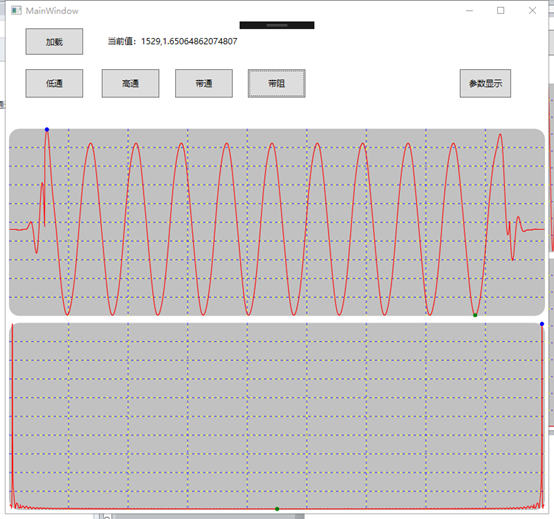10、总结：

1）基本能实现滤波功能，但是有很多参数以及算法需要再研究优化，仅提供一种思路；（未涉及到FIR的4种形式）

2）从效果来看，高通与带阻滤波后会出现很多高频的干扰（频段），而低通与带通没有，图中能看出，还需要进一步研究；

3）本文采用的是时域卷积的处理方式，其实也可以用差分方程来做，自己赖还没弄，处理完后都有数据点增多以及相移（线性）的问题，需要想办法优化；

更新：5）在使用时发现，高通滤波时若信号含有直流分量，则滤波结束后可能会引入低频噪声，将直流分量滤除后再进行滤波效果就会好很多；


展开全文C# FIR滤波器
• /****************************...** 程序名称：FIR低通滤波器 ** 程序描述：50阶FIR低通滤波器，通过频率2500Hz，截止频率3000Hz ** 性能提升： ** 程序版本：V1.0 ** 程序作者：syrchina ** 最后修改：2011年8月1日
/*******************************************************************************
** 程序名称：FIR低通滤波器
** 程序描述：50阶FIR低通滤波器，通过频率2500Hz，截止频率3000Hz
** 性能提升：
** 程序版本：V1.0
** 程序作者：syrchina
** 最后修改：2011年8月1日
*******************************************************************************/

#include <stdio.h>

/**********************************宏定义**************************************/
#define PI 3.1415926535
#define Size_Datas 64

/*******************************数据类型构造***********************************/
typedef float DataType;

int main(void)
{
DataType Xin[Size_Datas], Output=0, sum=0;
int n=0, i=0, nNow=0;
short int h = {
-35,   -219,   -503,   -494,     -8,    347,     19,   -348,     26,
404,   -103,   -485,    220,    579,   -398,   -678,    663,    774,
-1073,   -859,   1769,    925,  -3279,   -968,  10363,  17367,  10363,
-968,  -3279,    925,   1769,   -859,  -1073,    774,    663,   -678,
-398,    579,    220,   -485,   -103,    404,     26,   -348,     19,
347,     -8,   -494,   -503,   -219,    -35};
const int ORDER = sizeof(h)/sizeof(h);

for(i=0; i<Size_Datas; i++)//模拟输入
{
Xin[i] = ((sin(2*PI*i*2000/10000) + sin(2*PI*i*4000/10000)));
}
n = ORDER;

while (1)
{
//input new x[]
sum = 0;
for (i=0; i<ORDER; i++)
{
nNow = n - i;
if(nNow < 0)
{
nNow = Size_Datas + nNow;
}
sum = sum + h[i] * Xin[nNow];
}
Output = sum / 32767;
n = (n+1) & 0x3f;
//Output
}

return 0;
}
整理一下：

/*******************************************************************************
** 程序名称：FIR低通滤波器
** 程序描述：50阶FIR低通滤波器，通过频率2500Hz，截止频率3000Hz
** 性能提升：
** 程序版本：V1.0
** 程序作者：syrchina
** 最后修改：2011年8月1日
*******************************************************************************/

#include <stdio.h>

/**********************************宏定义**************************************/
#define PI 3.1415926535
#define Size_Datas 64

/*******************************数据类型构造***********************************/
typedef float DataType;

int main(void)
{
DataType Xin[Size_Datas], Output=0, sum=0;
int n=0, i=0, nNow=0;

// h[]参数使用matlab的FDAtool计算获得：50阶FIR低通滤波器，通过频率2500Hz，截止频率3000Hz
short int h = {
-35,   -219,   -503,   -494,     -8,    347,     19,   -348,     26,
404,   -103,   -485,    220,    579,   -398,   -678,    663,    774,
-1073,   -859,   1769,    925,  -3279,   -968,  10363,  17367,  10363,
-968,  -3279,    925,   1769,   -859,  -1073,    774,    663,   -678,
-398,    579,    220,   -485,   -103,    404,     26,   -348,     19,
347,     -8,   -494,   -503,   -219,    -35};
const int ORDER = sizeof(h)/sizeof(h);

for(i=0; i<Size_Datas; i++)//模拟输入, 64个原始数据
{
Xin[i] = ((sin(2*PI*i*2000/10000) + sin(2*PI*i*4000/10000)));
}

n = ORDER;
while (1)//循环演示
{
//input new x[]

sum = 0;
for (i=0; i<ORDER; i++)//FIR运算
{
nNow = n - i;
if(nNow < 0)
{
nNow = Size_Datas + nNow;
}
sum = sum + h[i] * Xin[nNow];
}
Output = sum / 32767;
n = (n+1) & 0x3f;// 0~63循环

//Output
}

return 0;
}



展开全文语言 c output float
• 基于C#的窗函数法低通FIR滤波器 摘要 基于Visual Studio 2015 开发环境，使用C#编程语言，运用窗函数法构造了FIR低通滤波器。并通过MATLAB对其滤波效果进行了试验。 由于凯泽窗的数学模型过于复杂，笔者能力有限...
基于C#的窗函数法低通FIR滤波器
摘要
基于Visual Studio 2015 开发环境，使用C#编程语言，运用窗函数法构造了FIR低通滤波器。并通过MATLAB对其滤波效果进行了试验。
由于凯泽窗的数学模型过于复杂，笔者能力有限，故无法在本文中给出凯泽窗的窗函数代码。
源代码
1、矩形窗函数
namespace Low_Pass_FIR_Fliter
{
class Boxcar
{
public int N = 0;
public Boxcar (double Wp, double Ws)
{
int i;
double n = (0.9 * 2 * Math.PI) / (Ws - Wp);//计算滤波器长度
for (i = 0; i < n; i++) ;
N = i;//得到滤波器长度
}
public double[] Get_Win()//返回N点矩形窗序列
{
int n;
double[] wd = new double[N];
for (n = 0; n < N; n++)
{
wd[n] = 1;
}
return wd;
}
}
}

2、三角窗函数
namespace Low_Pass_FIR_Fliter
{
class Bartlett
{
public int N = 0;
public Bartlett (double Wp, double Ws)
{
int i;
double n = (2.1 * 2 * Math.PI) / (Ws - Wp);//计算滤波器长度
for (i = 0; i < n; i++) ;
N = i;//得到滤波器长度
}
public double[] Get_Win()//返回N点三角窗序列
{
int n;
double[] wd = new double[N];
for (n = 0; n < N; n++)
{
if(n<=((N-1)/2))
{
wd[n] = (2 * n / (N - 1));
}
if(n > ((N - 1) / 2))
{
wd[n] = 2 - (2 * n / (N - 1));
}
}
return wd;
}
}

3、汉宁窗
namespace Low_Pass_FIR_Fliter
{
class Hanning
{
public int N = 0;
public Hanning(double Wp, double Ws)
{
int i;
double n = (3.1 * 2 * Math.PI) / (Ws - Wp);//计算滤波器长度
for (i = 0; i < n; i++) ;
N = i;//得到滤波器长度
}
public double[] Get_Win()//返回N点汉宁窗序列
{
int n;
double[] wd = new double[N];
for (n = 0; n < N; n++)
{
double b = Math.Cos((2 * Math.PI*n) / (N - 1));
double res = (0.5 - 0.5 * b);
wd[n] = res;
}
return wd;
}
}
}

4、汉明窗
namespace Low_Pass_FIR_Fliter
{
public class Hamming
{
public int N = 0;
public Hamming(double Wp,double Ws)
{
int i;
double n = (3.3 * 2 * Math.PI) / (Ws-Wp);//计算滤波器长度
for (i = 0; i < n; i++) ;
N = i ;//得到滤波器长度
}
public double [] Get_Win()//返回N点汉明窗序列
{
int n ;
double[] wd=new double[N];
for(n=0;n<N;n++)
{
double b = Math.Cos((2*n * Math.PI) / (N-1));
double res = (0.54 - 0.46 * b);
wd[n] = res;
}
return wd;
}
}
}

5、布莱克曼窗
namespace Low_Pass_FIR_Fliter
{
class Blackman
{
public int N = 0;
public Blackman (double Wp, double Ws)
{
int i;
double n = (5.5 * 2 * Math.PI) / (Ws - Wp);//计算滤波器长度
for (i = 0; i < n; i++) ;
N = i;//得到滤波器长度
}
public double[] Get_Win()//返回N点布莱克曼窗序列
{
int n;
double[] wd = new double[N];
for (n = 0; n < N; n++)
{
double a = Math.Cos((2 * n * Math.PI) / (N - 1));
double b = Math.Cos((4 * n * Math.PI) / (N - 1));
double res = (0.42 - 0.5 * a + 0.08 * b);
wd[n] = res;
}
return wd;
}
}
}

6、单位冲激响应
namespace Low_Pass_FIR_Fliter
{
class UnitImpulseReact
{
private double Wc;
private int alpha;
private int N;
public UnitImpulseReact(double Wp,double Ws,int N)//构造单位冲激响应
{
this.Wc = 0.5 * (Wp + Ws);//取近似截止频率
if (N % 2 != 0)
{
this.alpha = (N - 1) / 2;//计算相移常数
}
else
{
this.alpha = N / 2;
}
this.N = N;
}
public double[] Get_UIR()//获取单位冲激响应序列
{
double[] hd = new double[N];
for(int n=0;n<N;n++)
{
double numerator = Math.Sin(Wc * (n - alpha));
double denominator = Math.PI * (n - alpha);
if (n == alpha)//当n=α时，取极限
{
hd[n] = Wc / Math.PI;
}
else
{
hd[n] = numerator / denominator;
}
}
return hd;
}
}
}

使用MATLAB做正确性检验
大致思路：自定义一个15点序列，通过频谱图确定技术指标。分别通过MATLAB标准做法和自编程序对其进行滤波，若滤波结果大致一致，则可认为这个滤波器程序是正确的。
自定义序列 x[n]={ 16    12    2       -4     -1     1       -3     11    -27   18    15    -9     11    21    3} 该序列对应的频谱图为：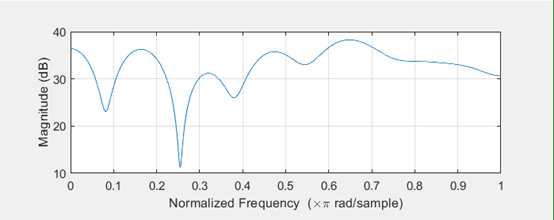选取通带边界频率ωp=0.2π，阻带边界频率ωs=0.3π，阻带衰减>30dB。由此，选择汉宁窗。通过MATLAB程序构成的低通滤波器b1的频谱图为：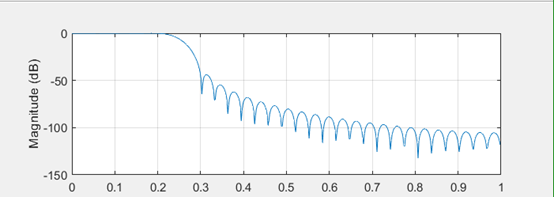滤波后的序列y的频率特性为（将>0dB的部分局部放大）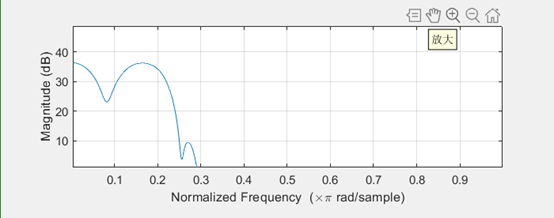同样的，在程序中基于预定的滤波器特性构建汉宁窗，并结合单位冲激响应得到滤波器的单位样值响应
using System;
using System.Collections.Generic;
using System.Linq;
using System.Text;
using System.IO;

namespace Low_Pass_FIR_Fliter
{
class Program
{
static void Main(string[] args)
{
Hanning win = new Hanning(0.2 * Math.PI, 0.3 * Math.PI);//构造汉宁窗
UnitImpulseReact UIR = new UnitImpulseReact(0.2 * Math.PI, 0.3 * Math.PI, win.N);//构造单位冲激响应
double[] hd = new double[win.N];
double[] w = new double[win.N];
double[] h = new double[win.N];
string[] res = new string[win.N];//定义数组
w = win.Get_Win();//获取窗函数序列
hd = UIR.Get_UIR();//获取单位冲激响应序列
for(int i=0;i<win.N;i++)
{
h[i] = w[i] * hd[i];//得到滤波器序列
}
for (int i = 0; i < win.N; i++)
{
res[i] = Convert.ToString(h[i]);//将滤波器序列点数转化成字符串形式，准备写入文件
}
File.WriteAllLines(@"C:\VS2015\C#Programe\Low-Pass_FIR_Fliter\Low-Pass_FIR_Fliter\sequence.txt", res);//写入文件，用于MATLAB的后续处理
}
}
}

通过程序获得的滤波器b2的频率特性为：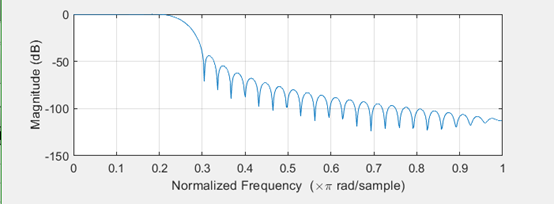程序滤波器滤波后的序列y1的频率特性为（同样将>0dB的部分局部放大）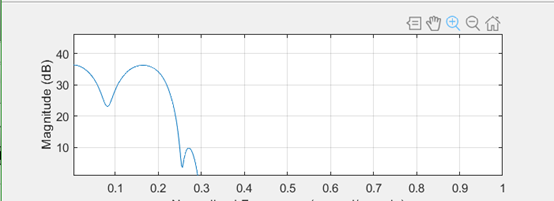通过波形比较可以认为程序生成的滤波器是成功的。


展开全文C# FIR低通滤波器 信号分析与处理
• c#实现的FIR数字滤波器，滤除50hz工频干扰；中值滤波实现滤除基线漂移C# 50HZ工频干扰 基线漂移 中值滤波
• 最近有一个需求，在C#中实现FIR滤波，网上查了些资料感觉FIR滤波使用的还算比较多，相关的原理也比较简单。参考下面在Python环境中实现FIR的博客，在C#的环境中实现了一遍。 ...
最近有一个需求，在C#中实现FIR滤波，网上查了些资料感觉FIR滤波使用的还算比较多，相关的原理也比较简单。参考下面在Python环境中实现FIR的博客，在C#的环境中实现了一遍。

https://blog.csdn.net/moge19/article/details/94495442（Python实现FIR带通滤波器）

一、FIR滤波器的设计，这里使用Matlab进行设计：

filterDesigner命令启动工具箱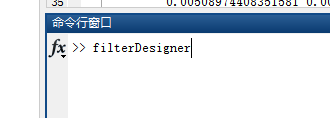设计FIR带通滤波，这里设计32阶，125-300赫兹，Hamming窗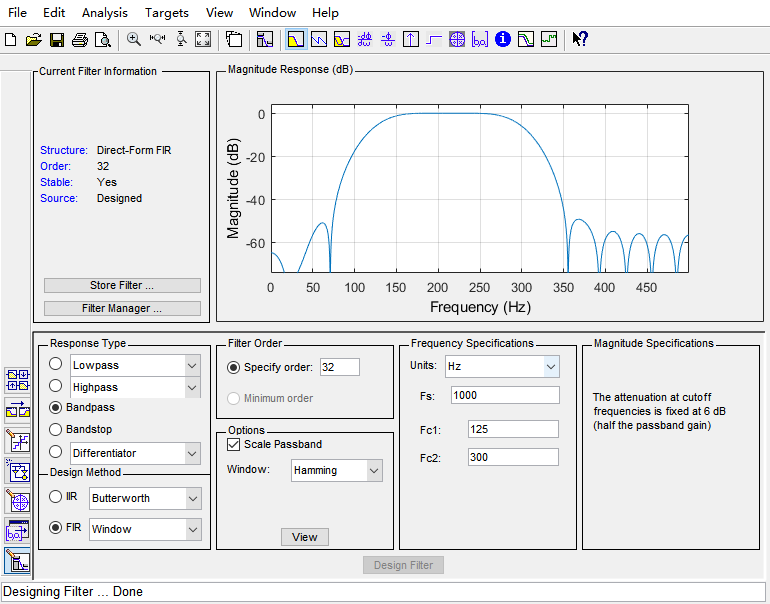输出设计的滤波器：这里面有3种不同的输出模式，你可以都输出看看，本质上都是同一个滤波器，只不过表现形式不同而已。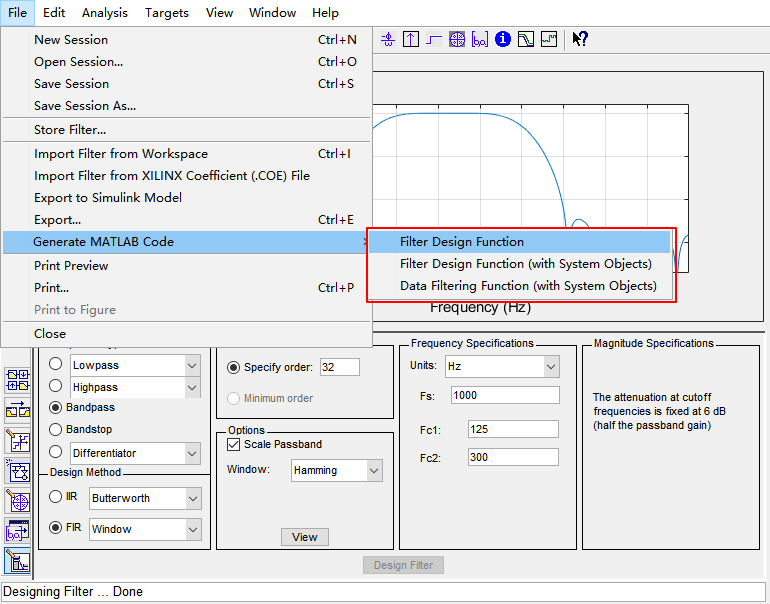matlab中的滤波效果验证（使用的是第一个选项生成的脚本，这里保存为FilteDesign1）：

第一列为时域数据，第二列为频域数据。

第一行为输入数据，第二行为FIR滤波器的结果，第三行为理想的滤波结果，图中可以看出滤波后的频谱和理想频谱还算接近，具体的效果需要根据实际情况调节阶数等滤波器的参数。

clc;clear;clf;close all

t = 0:0.001:0.05;
%原始数据
y = 0.7*sin(2*pi*30*t) + 1.8*sin(2*pi*160*t) + 5.1*sin(2*pi*330*t) ;
fft_y = abs(fft(y));
subplot(3,2,1);
plot(y)
subplot(3,2,2);
plot(fft_y)

%FIR滤波结果125-300
H = filter(FilteDesign1,y);
% H = filter(FilteDesign1,H');
fft_H = abs(fft(H));
subplot(3,2,3);
plot(H)
subplot(3,2,4);
plot(fft_H)
%理想结果
yH = 1.8*sin(2*pi*160*t);
fft_yH = abs(fft(yH));
subplot(3,2,5);
plot(yH)
subplot(3,2,6);
plot(fft_yH)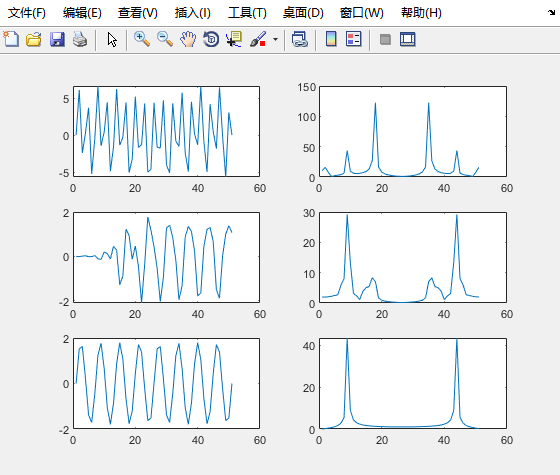二、FIR带通滤波的C#实现

个人理解滤波器的原理就是一句话：频域的乘积就是时域的卷积。实现滤波器的本质就是实现卷积运算，我们可以在matlab中获取上面设计滤波器的系数，在保存滤波器的时候选择第三个，在matlab函数中就可以获取到滤波器的系数h(i)。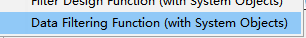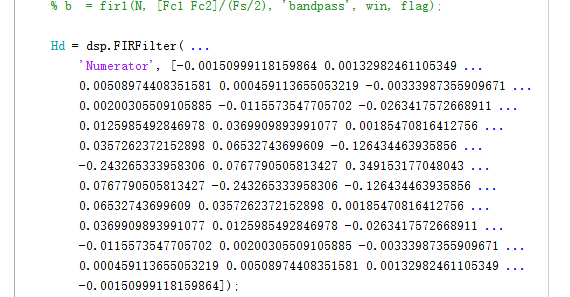C#的实现代码，filterParameters为对应的滤波器参数，其中CalcFIR为计算的核心部分，其输入参数为输入信号，返回值为滤波后的信号。

using System;
using System.Collections.Generic;
using System.Text;

namespace AlgorithmCSharp.Filter
{
class FilterFIR
{
public List<double> filterParameters { get; set; }

public FilterFIR(List<double> parameters)
{
filterParameters = parameters;
}

public double[] CalcFIR(double[] dataSerial)
{
int length = dataSerial.Length;
int order = filterParameters.Count;
double[] FIRResult = new double[length];

for(int i =0; i<length; i++)
{
double sum = 0;
if(i<order)
{
for(int j =0; j<i;j++)
{
sum += filterParameters[j] * dataSerial[i - j];
}
}
else
{
for(int j=0; j<order; j++)
{
sum += filterParameters[j] * dataSerial[i - j];
}
}
FIRResult[i] = sum;
}

return FIRResult;

}

}
}



展开全文C# Matlab
• 今天，我们也来装一装X，看看如何通过简单“写写画画”来设计一个FIR滤波器。 滤波器的概念相比大家都很熟悉了，一般按照频率特性可以分为低通、高通、带通及带阻滤波器，这是从输出特性来说的。 设计常规滤波器...
• 采用C Sharp编写的FIR滤波源代码，原创代码，内含产生带噪声的正弦波，FIR滤波器的卷积运算及滤波的源代码，Visual Studio2019
• 首先，先明白几个概念。通带，阻带，过渡带，通带纹波和阻带纹波分别是什么？看下图，  范围称为通带，对于允许... 通常的滤波器的设计，都会指明这几个参数，最后设计的滤波器，必须满足这几个参数。当然，这
• 利用Matlab设计滤波器，32阶的FIR带通滤波，在Matlab中获取相关参数，并且在C#环境中实现FIR滤波。
•滤波器设计
• 采用C Sharp(c#)编写的数字滤波器源代码，内含数字滤波器中卷积滤波的C#代码、FFT倒序的雷德算法、FFT完整代码、IIR数字滤波器的演示，FIR数字滤波器的演示、C#中Chart控件使用方法，原创代码，超经典。
• 任何一个数字滤波器都有幅频特性和相频特性，如果对于滤波不要求实时性，我们可以设计一种滤波器，使得它的相频特性始终为0，这种数字滤波器就称为零相移数字滤波器。 在Matlab中，零相移滤波器对应的函数名称为...
• 讲到图像处理的滤波器，就不得不先提图像中的低频分量和高频分量： 图像的频率代表了，图像颜色变化的剧烈程度。 低频分量：一幅图中，颜色变化缓慢的部分就叫做低频部分。通常低频是描述图像的主要部分，就像人的...
• -------------------------------------------------------------------------------------------------- 1.两种滤波器都是数字滤波器。...对于FIR滤波器，冲激响应在有限时间内衰减为零，其输出仅取决于当前
• 本文将以FIR滤波器的设计为主题，详细介绍如何把一个设计“流水线化”，并进行时序分析体会流水线设计带来的好处。 流水线 这里先用通俗易懂的语言描述一下流水线设计思想。假设小A要从成都到哈尔滨旅游，如果...pipeline
• 其中的低通滤波器的时域表达式为 y[n] = 2*y[n-1] - y[n-2] + x[n] - 2*x[t-24 ms] + x[t-48 ms] 请问这个截止频率是多少？ { static signed long y1 = 0, y2 = 0 ; static signed long d0,d1,d2,...c语言
• 滤波器的作用是允许某些频率的正弦信号基本无衰减的通过（增益为 1，频域 0dB），同时对另外某些频率的正弦信号起到衰减作用（增益在 0.707 以下，频域-3dB 以下）。 当然，滤波器对通过的正弦信号会产生一定的相移...
• 而一阶RC滤波器容易实现。但是网上资料往往没有详细的推导。因此在这里把笔记记下。本文的优势是比较详细，参数配置都有公式依据。 目录 1、一阶RC低通滤波器的算法实现 1.1 算法推导 1.2 波特图 1.3 用C语言实现 2...